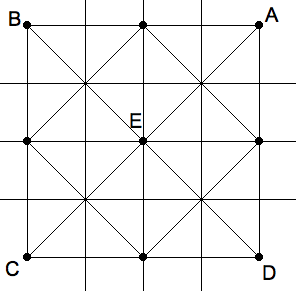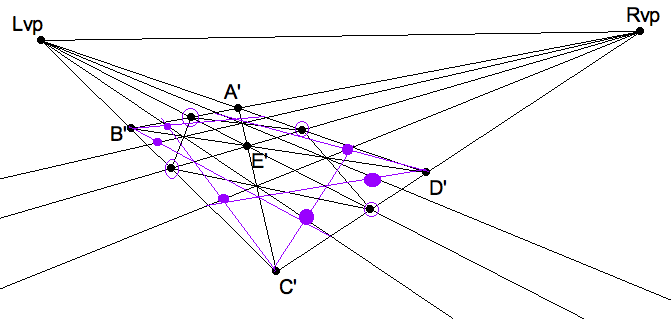As we have seen in the previous lesson on perspective rulers, subdividing and multiplying lengths in perspective can be done by applying the rules of cross-ratios. There is, however, nothing like a "perspective compass" for drawing circles, or circular arcs. For this purpose, perpectivist have developed other techniques, one of which is the subject of this lessson.

## The method of diagonals

Suppose we have a figure inside a rectangular frame, for example a circle inscribed in a square, and we wish to draw this figure into a perspective view of the rectangle. In perspective, the rectangle will be a convex quadrilateral, with two vanishing points. Let the corners of the Euclidean square be $ABCD$ and the corresponding corners of the perspective square in the picture plane be labelled $A'B'C'D'$. If we draw the diagonals of both quadrilaterals, then to intersect at $E$ and $E'$ respectively, then $E'$ is the perspective image of $E$, the midpoint of the square. To divide the square into four quarters, we draw lines through $E$ that are parallel to the sides of the square. We know how to draw perspective parallels, namely by connecting $E'$ with each of the two vanishing points. We can continue this process indefinitely, and obtain a division of the original square into grid of 4, 16, 64, 256 … equal squares. And, at the same time, its perspective image in the picture plane. Such a division "by halves" is called a binary grid.Orthogonal four-by-four grid.

## Binary grids for sketching

Using a pair of binary grids, one orthographic and the other in perspective, we can transfer an arbitrary figure in the former to the latter by approximating the situation in each pair of corresponding squares. You can even imagine copying from one perspective frame into another, at least approximately. Duerer’s picture of the artist using Alberti’s Veil is an example of this technique.Four-by-four grid in perspective.

Now consider the circle inscribed into a square. Diagonalize once to obtain the cross of a cartesian coordinate system with origin the center of the Euclidean square $ABCD$. Where the axes intersect the sides of the square are four points on the circles. Subdivide once more to obtain a grid of 16 squars. The 4 additional lines have equations $x= \pm \frac{1}{2}, y = \pm \frac{1}{2}$. Imagine, but do not draw, two further binary subdivisions, so that lines like $y=\frac{7}{8}$ have their perspective counterparts in the picture plane. By the Pythagorean theorem, the point 8 points $( \pm \frac{1}{2}, \pm \frac{7}{8}), ( \pm \frac{7}{8}, \pm \frac{1}{4})$ all are very nearly on the unit circle because

 $\frac{1}{2}^2+\frac{7}{8}^2=\frac{4}{8}^2+\frac{7}{8}^2=\frac{16+49}{64}=\frac{65}{64} ~ 1$

## Shooting the corners

Now, these eight "seven-eighths" points can be located already on the 2nd grid (the one with 16 squares) with the help of four diagonals of 1 by 4 rectangles. Consider an orthographic rectangle in the proportion 4:1. The diagonal starting at the upper left corner cuts the first vertical quarter line at its $\frac{3}{4}$ point, the second at its midtpoint etc. Only the first crossing is of interest, because $\frac{1}{2}+\frac{3}{4}\frac{1}{2} = \frac{7}{8}$.Each corner shoots twice across 4-by-1 rectangles to find 8 more points.

So, in perspective, after subdividing the perspective square once, we obtain 4 of the 12 points on the perspective circle. Subdividing again, and shooting the corners we could obtain up to 8 more reference points on the circle. Of course, only those in the largest quadrilaterals are important, if you can trust yourself to get along with just 8 points on the circle.The back corner is too small to shoot. The 10 points suffice.

In practice, one can usually skip the back seven-eighths points and still sketch a tolerably good circle in perspective.